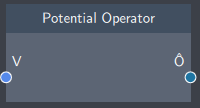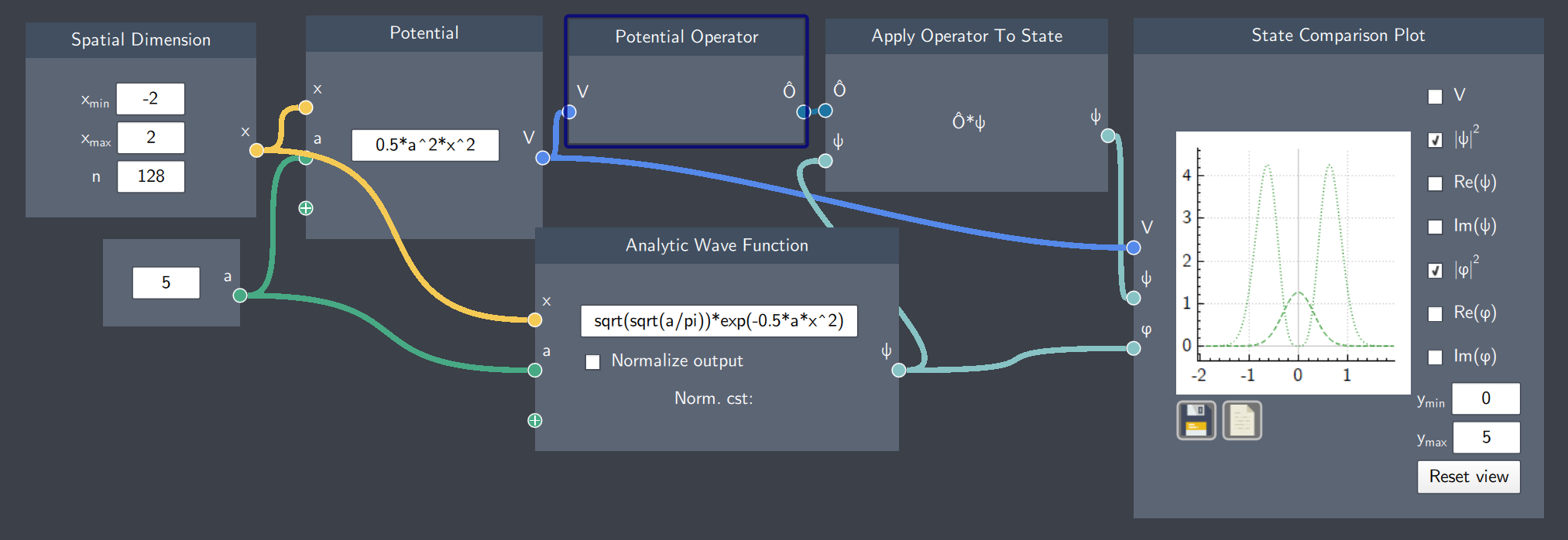# Potential Operator

Description

The Potential Operator is the quantum mechanical operator that changes a potential function to an operator.## Input

• Potential (V): It inputs a function defined in the Potential node.

## Content

Transformation of a potential function into a potential energy operator.

## Output

• Potential Operator: The resulting potential energy operator $\hat{V}$ which can be applied to a state.

## Example

In the example below, the Potential Operator node inputs the harmonic oscillator potential function and applies the operation to a Gaussian function which results in a new function. The magnitudes of both the Gaussian and the resulting function after operator application are shown on the plot.